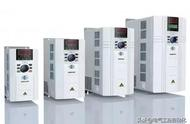# 西门子MM440变频器参数设置问题P0100=0

P0205=0

P0300=1

P0304=400

P0305=3.7

P0307=1.5

P0310=50

P0311=1425

P0700=1BOP面板手动操作方式

P1000=1BOP面板手动操作方式

P1080=0

P1082=50

P1120=10

P1121=10

P1135=5

P1300=20

P3900=1变频器显示BUSY大约10秒后，完成快速调试。

P0756=2

P0757=4

P0758=0

P0759=20

P0760=100%对应50Hz，80%对应40Hz，70%对应35Hz。

P0761=4.00避免变频器由于4-20mA控制信号的飘移，低于4mA时，造成变频器反转，对低于4mA的控制信号，变频器均认为是4mA，且不会反转。

P0700=2外部自动控制方式

P1000=2外部自动控制方式